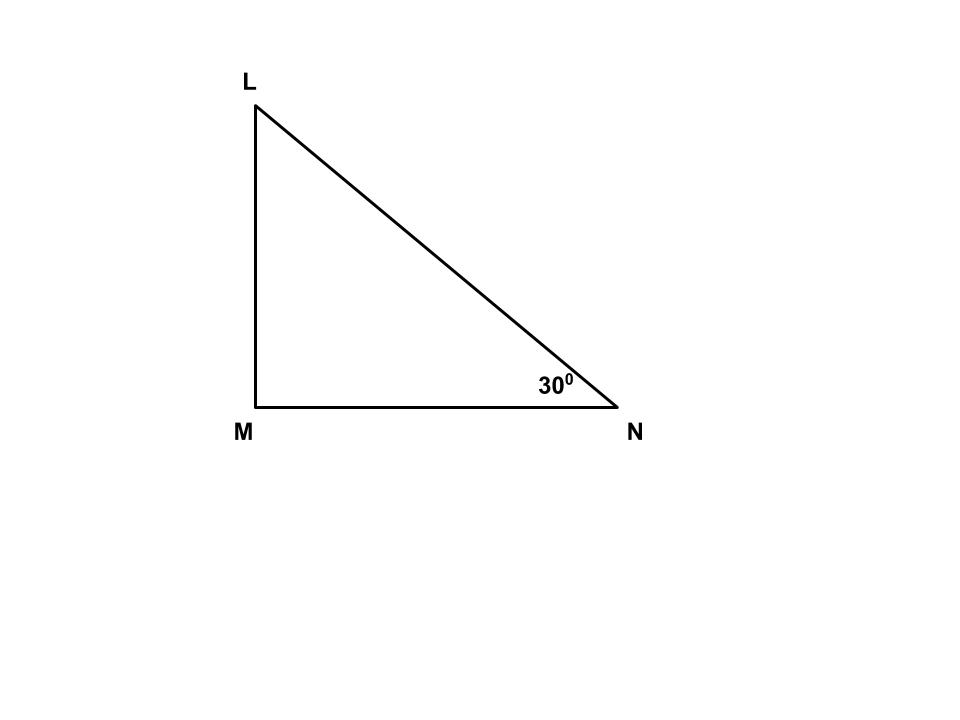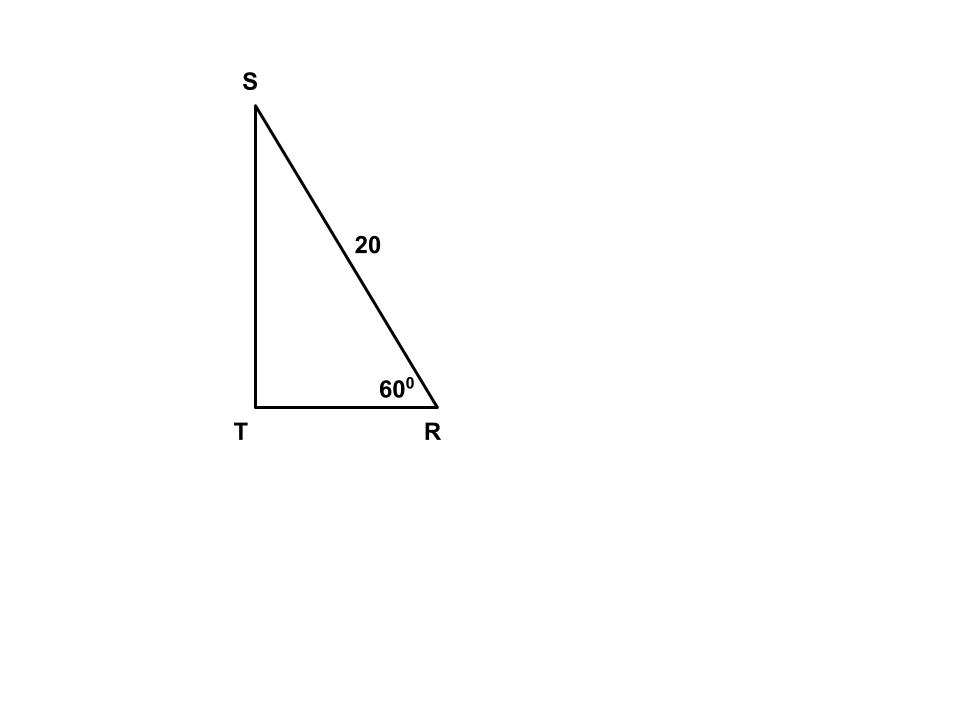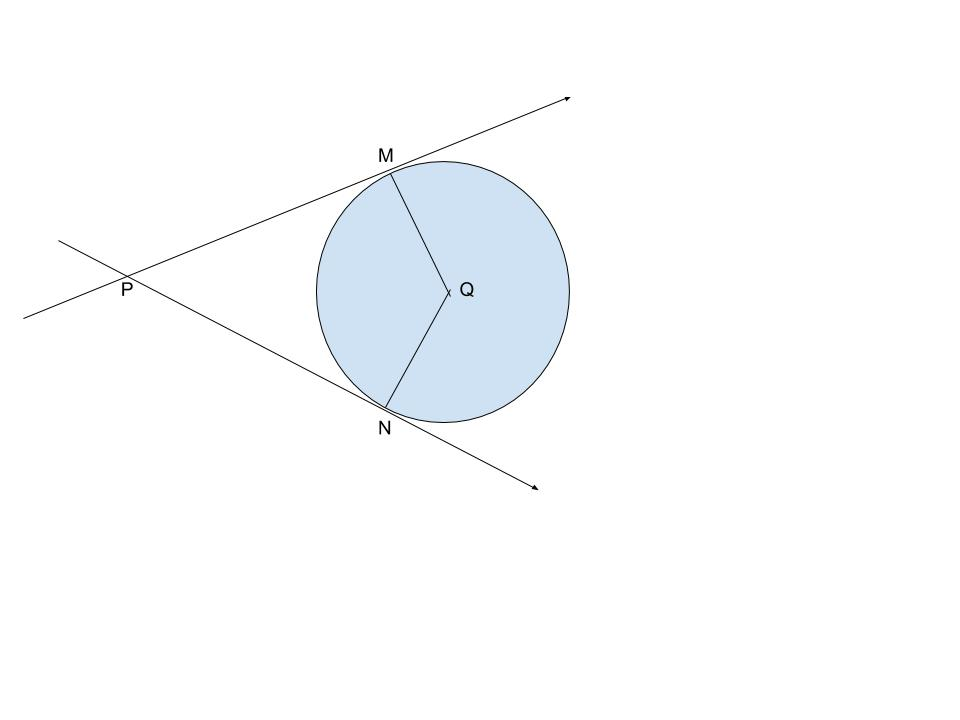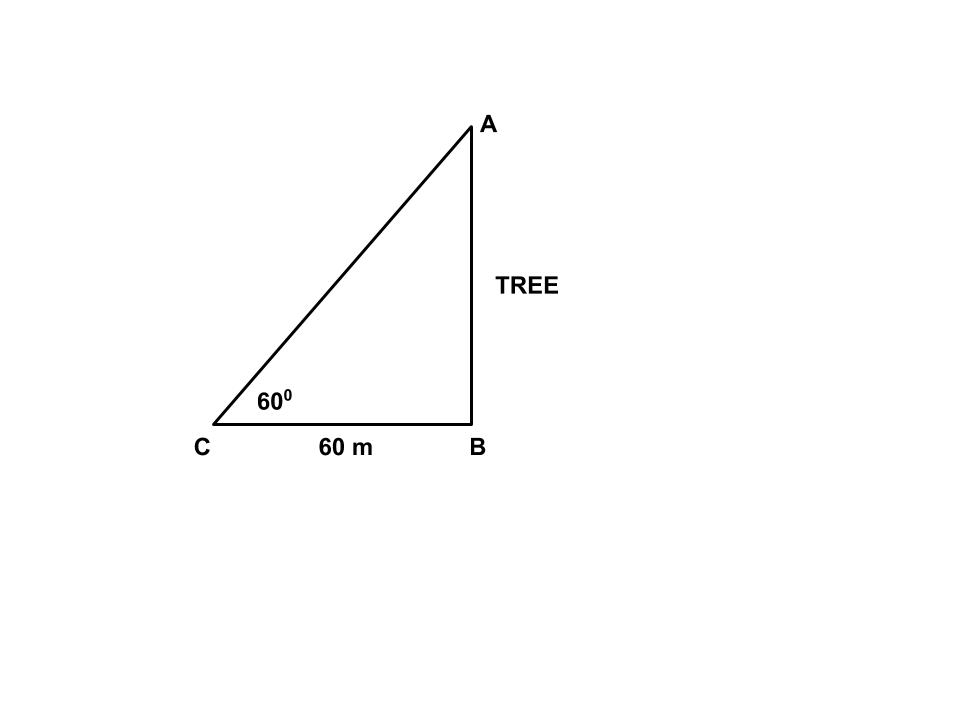SSC BOARD PAPERS IMPORTANT TOPICS COVERED FOR BOARD EXAM 2024

### GENERAL MATHS GEOMETRY GEOMETRY PRELIMINARY PAPER 2016

OMTEX CLASSES THE HOME OF SUCCESS.

GENERAL MATHS GEOMETRY
GEOMETRY PRELIMINARY PAPER 2016

1. Solve any five of the sub – questions: 

(i) If sin A = 2/7 then find cosec A.
(ii) Draw segment AB of length 6.5 cm. Draw the perpendicular bisector of segment AB.
(iii) Draw ∠PQR of measure 850 and bisect it.
(iv) Find the surface are of the vertical faces of cuboid. l = 5, b = 3, h = 4.
(v) If diameter of a circle is 18 cm then find the radius of the circle.
(vi) If sin P = 4/5 and tan P = 4/3 then find cos P.

2. Solve any four sub - questions: 

(i) In the following figure, ∆ LMN is a right angled triangle, where ∠M = 900. ∠ N = 300. Write the ratios of sin 300 and tan 600.(ii) Find the value of the following: sin 450 x cos 450 - sin 300.
(iii) Draw any right angled triangle. Draw perpendicular bisector of the hypotenuse.
(iv) For a cylinder radius is 7 cm and height is 15 cm. Find its total surface area. ( = 22/7)
(v) Find the volume of sphere of diameter 6 cm. ( =3.14)
(vi) ∆ PQR ~ ∆ ZXY AND PQ = 12, QR = 8, PR = 15,  ZX = 18, Find XY and YZ.

3. Solve any three sub - questions: 
(i) The ratio of areas of two triangles with equal heights is 3:4. The base of the smaller triangle is 15. Find the corresponding base of the larger triangle.

(ii) If the sides of a rectangle are 9 cm and 40 cm. Find the length of its diagonal.
(iii) Using the information given in the following figure, find ST and TR.(iv) In the following figure, Q is the centre of a circle and PM, PN are tangent segments to the circle. If  ∠ MPN = 500, find ∠ MQN.(v) A boy is at a distance of 60 metres from a tree makes an angle of elevation of 600 with the top of the tree. What is the height of the tree?(vi) Prove that ‘the opposite angles of a cyclic quadrilateral are supplementary”.

4. Solve any two sub – questions: 
(i) If a line parallel to one side of a triangle intersects the other two sides in two distinct points, then the other two sides are divided in the same ratio by it.
(ii) Draw the circumcircle of Triangle PQR in which PQ = 5 cm, QR = 5 cm and Angle PQR = 750.